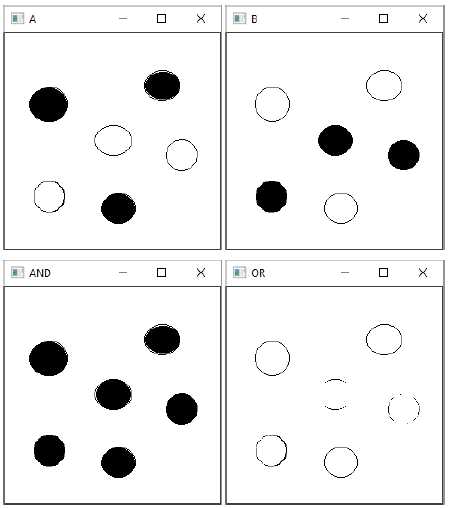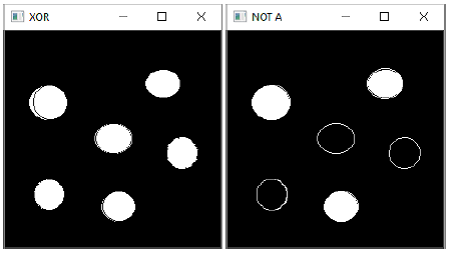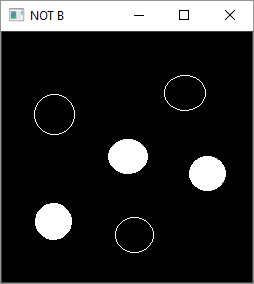# OpenCV Python - Bitwise Operations

Bitwise operations are used in image manipulation and for extracting the essential parts in the image.

Following operators are implemented in OpenCV −

• bitwise_and
• bitwise_or
• bitwise_xor
• bitwise_not

## Example 1

To demonstrate the use of these operators, two images with filled and empty circles are taken.

Following program demonstrates the use of bitwise operators in OpenCV-Python −

import cv2
import numpy as np

dest1 = cv2.bitwise_and(img2, img1, mask = None)
dest2 = cv2.bitwise_or(img2, img1, mask = None)
dest3 = cv2.bitwise_xor(img1, img2, mask = None)

cv2.imshow('A', img1)
cv2.imshow('B', img2)
cv2.imshow('AND', dest1)
cv2.imshow('OR', dest2)
cv2.imshow('XOR', dest3)
cv2.imshow('NOT A', cv2.bitwise_not(img1))
cv2.imshow('NOT B', cv2.bitwise_not(img2))

if cv2.waitKey(0) & 0xff == 27:
cv2.destroyAllWindows()


## Output## Example 2

In another example involving bitwise operations, the opencv logo is superimposed on another image. Here, we obtain a mask array calling threshold() function on the logo and perform AND operation between them.

Similarly, by NOT operation, we get an inverse mask. Also, we get AND with the background image.

Following is the program which determines the use of bitwise operations −

import cv2 as cv
import numpy as np

rows,cols,channels = img2.shape
roi = img1[0:rows, 0:cols]
img2gray = cv.cvtColor(img2,cv.COLOR_BGR2GRAY)
ret, mask = cv.threshold(img2gray, 10, 255, cv.THRESH_BINARY)
# Now black-out the area of logo

# Take only region of logo from logo image.
# Put logo in ROI